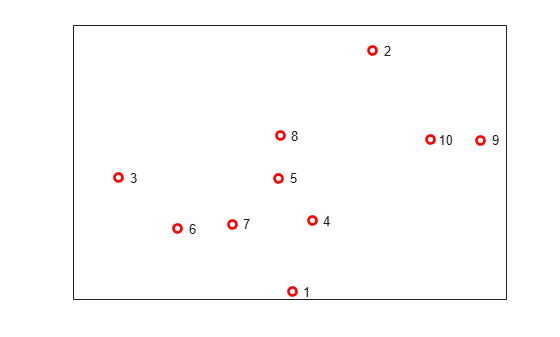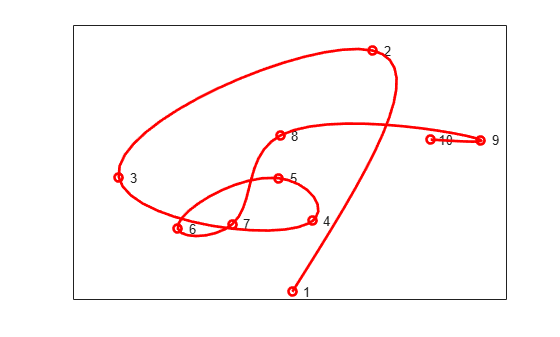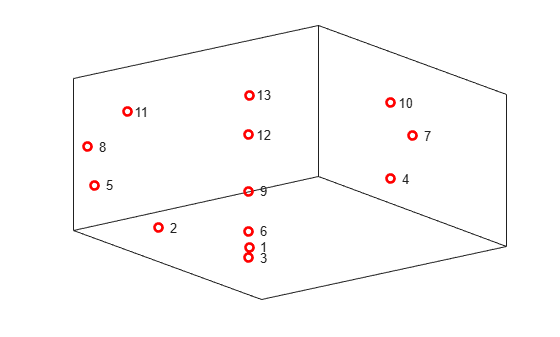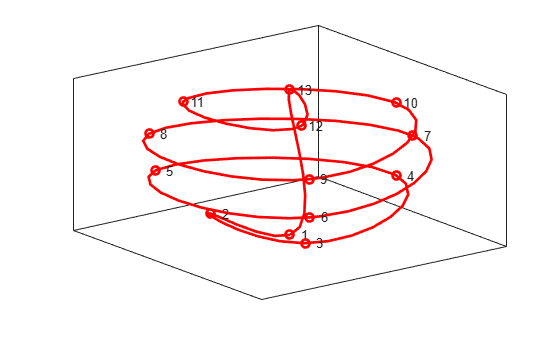Documentation

### This is machine translation

Mouseover text to see original. Click the button below to return to the English version of the page.

Note: This page has been translated by MathWorks. Click here to see
To view all translated materials including this page, select Country from the country navigator on the bottom of this page.

## Constructing Spline Curves in 2D and 3D

This example shows how to use the `cscvn` command from Curve Fitting Toolbox™ to construct cubic spline curves in two and three dimensions.

### Selecting the Points

This example will show how to draw a smooth curve through a list of points, in the order in which they occur. First, we select some random points in the plane, and store them in a matrix, one point per column.

```npts = 10; xy = [randn(1,npts); randn(1,npts)]; plot(xy(1,:),xy(2,:),'ro','LineWidth',2); text(xy(1,:), xy(2,:),[repmat(' ',npts,1), num2str((1:npts)')]) ax = gca; ax.XTick = []; ax.YTick = [];```### Connecting the Points

Next, construct the curve using the `cscvn` command and plot it using `fnplt`.

```hold on fnplt(cscvn(xy),'r',2) hold off```You could also use the `getcurve` command if you wanted to input the list of points interactively.

### 3-D Spline Curves

It's just as easy to create spline curves in three dimensions. This time, we'll do something less random. First, we generate the points.

```npts = 13; t = linspace(0,8*pi,npts); z = linspace(-1,1,npts); omz = sqrt(1-z.^2); xyz = [cos(t).*omz; sin(t).*omz; z]; plot3(xyz(1,:),xyz(2,:),xyz(3,:),'ro','LineWidth',2); text(xyz(1,:),xyz(2,:),xyz(3,:),[repmat(' ',npts,1), num2str((1:npts)')]) ax = gca; ax.XTick = []; ax.YTick = []; ax.ZTick = []; box on```### Connecting the Points

Here is the 3D spline curve through these points provided by `cscvn`. By appending the first point to the end of the list, we get a smooth closed curve.

```hold on fnplt(cscvn(xyz(:,[1:end 1])),'r',2) hold off```